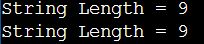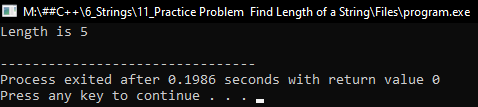# How to Find Length of a String in C++

## How to Find the Length of a String in C++:

In this article, I am going to discuss How to Find the Length of a String in C++ Language with Examples. Please read our previous article, where we discussed String Iterator in C++ with examples.

##### Methods to find the length of the string in C++
1. Using size function: The size method returns the length of the string, in terms of bytes.
2. Using length function: The length method returns the length of the string, in terms of bytes.
3. Using the C library function strlen() method: The C library function size_t strlen(const char *str) computes the length of the string str up to, but not including the terminating null character.
4. Using while loop: Using the traditional method, initialize the counter equals 0 and increment the counter from starting of the string to the end of the string (terminating null character).
5. Using for loop: To initialize the counter equals 0 and increment the counter from starting of string to end of the string (terminating null character).
##### Method1: Find the length of a string by using size() and length() functions

We can get the length of a string in C++ by using the size() and length() functions. The size() and length() functions are just synonyms and they both do the same thing.

```#include <iostream>
using namespace std;
int main()
{
string str = "Learn C++";
cout << "String Length = " << str.size ();
cout << "\nString Length = " << str.length ();
return 0;
}```
###### Output:##### Length of C-style string using strlen() function

To get the length of a C-string string, strlen() function is used in the C++ programming language. The C library function size_t strlen(const char *str) computes the length of the string str up to, but not including the terminating null character.

```#include <iostream>
#include <cstring>
using namespace std;

int main()
{
char str[] = "Lerarn C++ Programming Language";
cout << "String Length = " << strlen (str);
return 0;
}```

Output: String Length = 31

##### Method1: By using for loop to find the length of a string

In the below program we use for loop to Find the Length of a given String using C++ Language.

```#include <iostream>
#include <string.h>
using namespace std;
int main()
{
string str = "World";
int count = 0;
for (int i = 0; str[i] != '\0'; i++)
{
count++;
}
cout << "Length is " << count << endl;
return 0;
}
```
###### Output:##### Method2: By using iterator

In the following program, we use the string iterator to Find the Length of a given String using C++ Language.

```#include <iostream>
#include <string.h>
using namespace std;
int main()
{
string str = "World";
string::iterator it;
int count = 0;
for (it = str.begin (); it != str.end (); it++)
{
count++;
}
cout << "Length is " << count << endl;
return 0;
}
```
###### Output: